Mathematical Models in Engineering

Published: 30 June 2022

# Improved algorithm for a system with different stiffness for positive and negative displacements

K. Ragulskis1
B. Spruogis2
A. Matuliauskas3
V. Mištinas4
L. Ragulskis5
1Kaunas University of Technology, K. Donelaičio str. 73, LT-44249, Kaunas, Lithuania
2, 3, 4Department of Mobile Machinery and Railway Transport, Faculty of Transport Engineering, Vilnius Gediminas Technical University, Plytinės str. 27, LT-10105, Vilnius, Lithuania
5Department of Systems Analysis, Faculty of Informatics, Vytautas Magnus University, Vileikos str. 8, LT-44404, Kaunas, Lithuania
Corresponding Author:
K. Ragulskis
Views 19

#### Abstract

In the process of investigation of dynamics of elements of robots, systems with different values of stiffness for positive and negative displacements play an important role. This change of the value of stiffness has an effect to the results of numerical calculation of dynamics of the system. For more precise investigation of dynamics a special numerical procedure is proposed in the paper. Numerical results for two values of time steps: large and medium ones, are presented for two cases: without application of the proposed procedure and with application of it. Comparison of the presented results indicates advantages of the proposed numerical procedure.

## 1. Introduction

In the process of investigation of dynamics of elements of robots, systems with different values of stiffness for positive and negative displacements play an important role. This change of the value of stiffness has an effect to the results of numerical calculation of dynamics of the system.

For more precise investigation of dynamics a special numerical procedure is proposed in the paper. Numerical results for two values of time steps: large and medium ones, are presented for two cases: without application of the proposed procedure and with application of it.

Comparison of the presented results indicates advantages of the proposed numerical procedure.

Resonances in nonlinear systems are described in . Systems with impacts are investigated in . Stabilisation of dynamical systems is presented in . Impacts in vibrating systems are investigated in . Periodic orbits are analysed in . Vibro-impact energy sink is investigated in . Particle impact with a wall is presented in . Frequencies of a multibody system are analysed in . Pendulum and its dynamics are investigated in . Piecewise linearity is analysed in . Resonant zones are investigated in . Sommerfeld effect is presented in . Isolated resonances are investigated in .

First the model of the system with a single degree of freedom and harmonic excitation is described. Then the proposed procedure for improved calculation of dynamics is presented. Graphical results without application of the proposed procedure and with it are presented and mutually compared.

## 2. Model of the system with different values of stiffness for positive and negative displacements

The investigated system has a single degree of freedom and is described by the following differential equation:

1
$\stackrel{¨}{x}+2h\stackrel{˙}{x}+{p}^{2}x=-f\mathrm{s}\mathrm{i}\mathrm{n}\omega t,$

where $x$ is the displacement of the system, $h$ is the coefficient of viscous damping, ${p}^{2}$ is the nonlinear coefficient of stiffness, $f$ is the amplitude of harmonic excitation, $\omega$ is the frequency of harmonic excitation, $t$ is the time and the upper dot denotes differentiation with respect to it.

It is assumed that the nonlinear stiffness has the following form:

2

where ${p}_{1}$ and ${p}_{2}$ are constant values.

Further the force of stiffness is denoted as:

3
$P={p}^{2}x.$

## 3. Improved procedure for calculation of dynamics of the system with different values of stiffness for positive and negative displacements

Further the following notation is used: $T$ denotes the time step, the subscript $0$ denotes the value at the beginning of a time step and the subscript $T$ denotes the value at the end of a time step.

It is assumed that:

4

If the following conditions are satisfied:

5
${x}_{T}>0,$

and:

6
${x}_{0}<0,$

or if the following conditions are satisfied:

7
${x}_{T}<0,$

and:

8
${x}_{0}>0,$

then the reduced time step is used in the process of calculations:

9
${T}_{r}=-\frac{{x}_{0}}{{x}_{T}-{x}_{0}}T.$

Then it is assumed that:

10
${x}_{{T}_{r}}=0,$
11
${\stackrel{˙}{x}}_{{T}_{r}}={\stackrel{˙}{x}}_{0}+\left({\stackrel{˙}{x}}_{T}-{\stackrel{˙}{x}}_{0}\right)\frac{{T}_{r}}{T},$
12
${\stackrel{¨}{x}}_{{T}_{r}}={\stackrel{¨}{x}}_{0}+\left({\stackrel{¨}{x}}_{T}-{\stackrel{¨}{x}}_{0}\right)\frac{{T}_{r}}{T}.$

## 4. Investigation of dynamics of the system with different values of stiffness for positive and negative displacements

The following parameters of the investigated system with different values of stiffness for positive and negative displacements were assumed:

13

Zero initial conditions were assumed:

14

Results for two values of a time step are presented: large time step:

15
$T=\frac{2\pi }{\omega 40},$

and medium time step:

16
$T=\frac{2\pi }{\omega 80}.$

### 4.1.1. Conventional procedure for calculation of dynamics

Displacement, velocity, acceleration, and velocity multiplied by acceleration as functions of time are presented in Fig. 1.

Fig. 1Dynamics of the system with different values of stiffness for positive and negative displacements when ω=1, f= 1, h= 0.1, p1= 1, p2= 2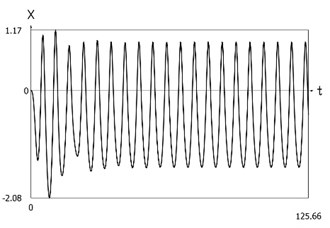a) Displacement as function of time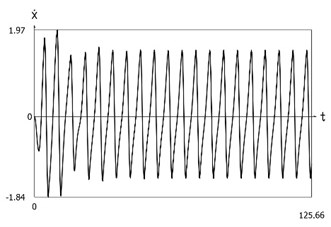b) Velocity as function of time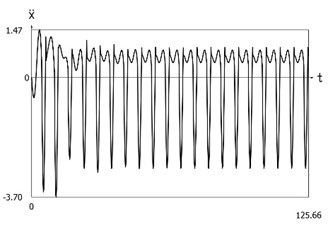c) Acceleration as function of time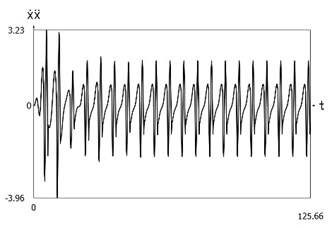d) Velocity multiplied by acceleration as function of time

Phase trajectories of motion of the investigated system are presented in Fig. 2.

Force of stiffness as function of displacement is shown in Fig. 3.

Fig. 2Phase trajectories of the system with different values of stiffness for positive and negative displacements when ω=1, f= 1, h= 0.1, p1= 1, p2= 2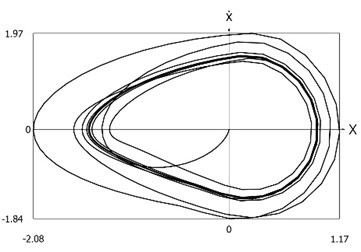a) Velocity as function of displacement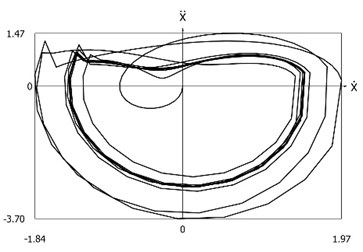b) Acceleration as function of velocity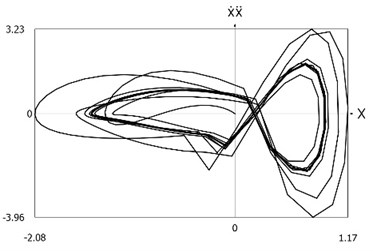c) Velocity multiplied by acceleration as function of displacement

Fig. 3Force of stiffness as function of displacement of the system with different values of stiffness for positive and negative displacements when ω=1, f= 1, h= 0.1, p1= 1, p2= 2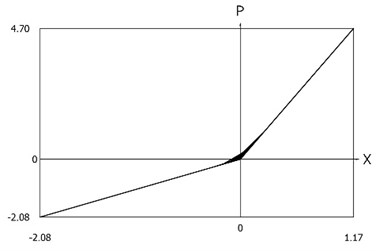### 4.1.2. Improved procedure for calculation of dynamics

Displacement, velocity, acceleration, and velocity multiplied by acceleration as functions of time are presented in Fig. 4.

Phase trajectories of motion of the investigated system are presented in Fig. 5.

Force of stiffness as function of displacement is shown in Fig. 6.

From the presented results it is seen that the improved procedure for calculation of dynamics has advantages, which are seen from the comparison of corresponding graphical representations. This is especially evident from the comparison of corresponding drawings involving accelerations as well as from the comparison of representations of force of stiffness as function of displacement in the vicinity of the origin of the coordinate system.

Fig. 4Dynamics of the system with different values of stiffness for positive and negative displacements when ω=1, f= 1, h= 0.1, p1= 1, p2= 2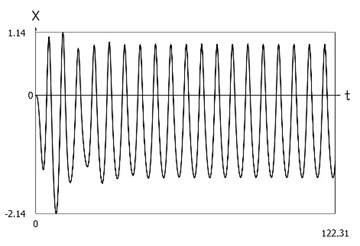a) Displacement as function of time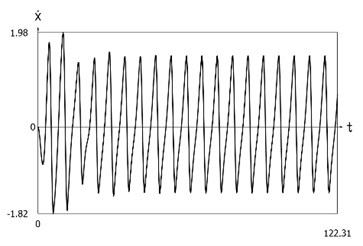b) Velocity as function of time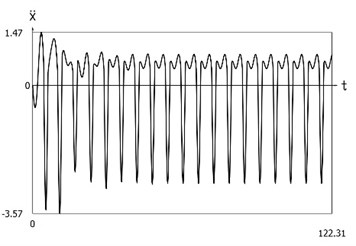c) Acceleration as function of time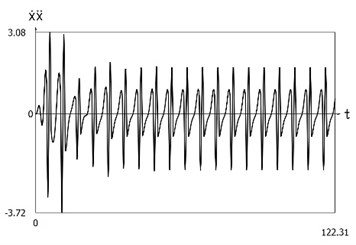d) Velocity multiplied by acceleration as function of time

Fig. 5Phase trajectories of the system with different values of stiffness for positive and negative displacements when ω=1, f= 1, h= 0.1, p1= 1, p2= 2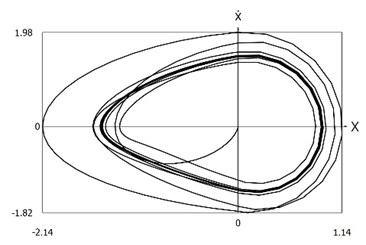a) Velocity as function of displacement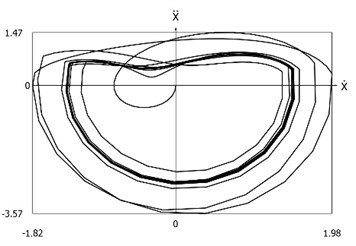b) Acceleration as function of velocity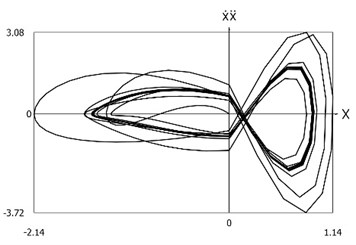c) Velocity multiplied by acceleration as function of displacement

Fig. 6Force of stiffness as function of displacement of the system with different values of stiffness for positive and negative displacements when ω=1, f= 1, h= 0.1, p1= 1, p2= 2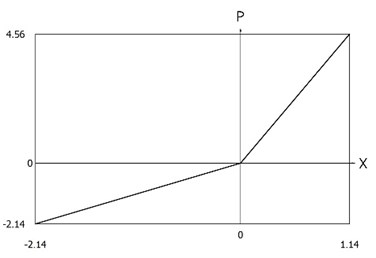### 4.2.1. Conventional procedure for calculation of dynamics

Displacement, velocity, acceleration, and velocity multiplied by acceleration as functions of time are presented in Fig. 7.

Fig. 7Dynamics of the system with different values of stiffness for positive and negative displacements when ω=1, f= 1, h= 0.1, p1= 1, p2= 2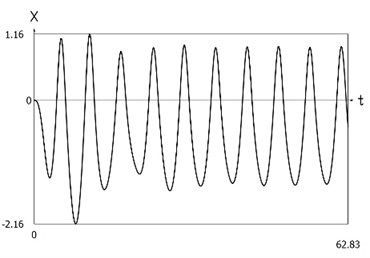a) Displacement as function of time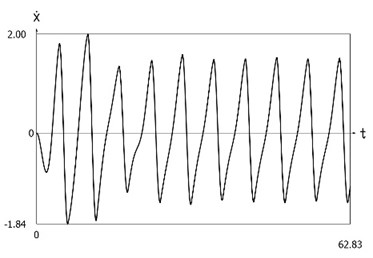b) Velocity as function of time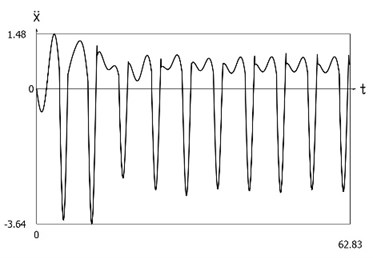c) Acceleration as function of time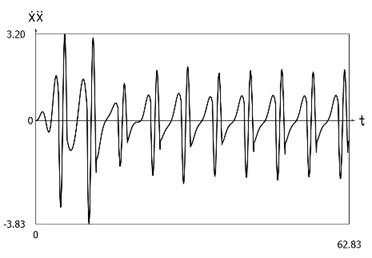d) Velocity multiplied by acceleration as function of time

Phase trajectories of motion of the investigated system are presented in Fig. 8.

Force of stiffness as function of displacement is shown in Fig. 9.

Fig. 8Phase trajectories of the system with different values of stiffness for positive and negative displacements when ω=1, f= 1, h= 0.1, p1= 1, p2= 2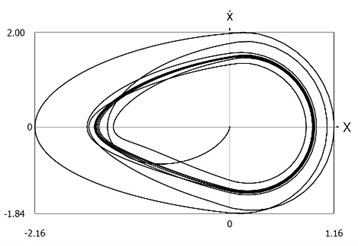a) Velocity as function of displacement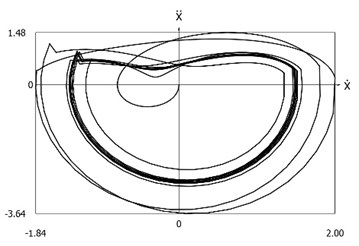b) Acceleration as function of velocity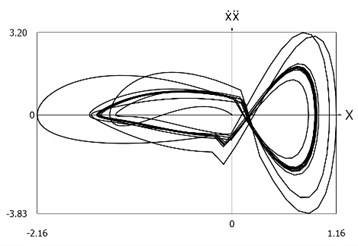c) Velocity multiplied by acceleration as function of displacement

Fig. 9Force of stiffness as function of displacement of the system with different values of stiffness for positive and negative displacements when ω=1, f= 1, h= 0.1, p1= 1, p2= 2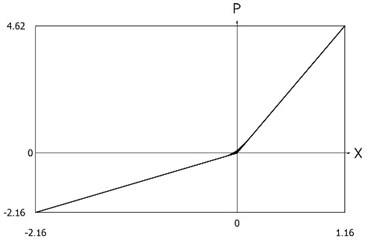### 4.2.2. Improved procedure for calculation of dynamics

Displacement, velocity, acceleration, and velocity multiplied by acceleration as functions of time are presented in Fig. 10.

Phase trajectories of motion of the investigated system are presented in Fig. 11.

Force of stiffness as function of displacement is shown in Fig. 12.

From the presented results it is seen that the improved procedure for calculation of dynamics has advantages, which are seen from the comparison of corresponding graphical representations. This is especially evident from the comparison of corresponding drawings involving accelerations as well as from the comparison of representations of force of stiffness as function of displacement in the vicinity of the origin of the coordinate system.

The presented graphical representations show the advantages of the improved procedure for calculation of dynamics of the system with different values of stiffness for positive and negative displacements.

Fig. 10Dynamics of the system with different values of stiffness for positive and negative displacements when ω=1, f= 1, h= 0.1, p1= 1, p2= 2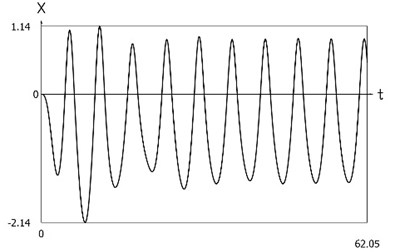a) Displacement as function of time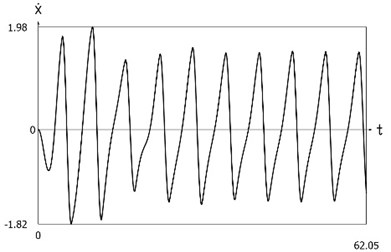b) Velocity as function of time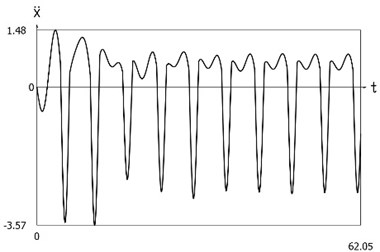c) Acceleration as function of time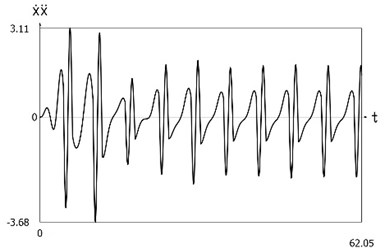d) Velocity multiplied by acceleration as function of time

Fig. 11Phase trajectories of the system with different values of stiffness for positive and negative displacements when ω=1, f= 1, h= 0.1, p1= 1, p2= 2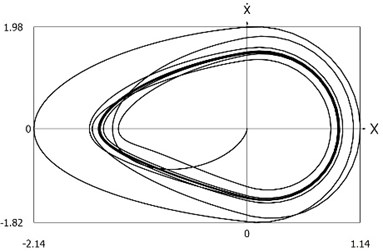a) Velocity as function of displacement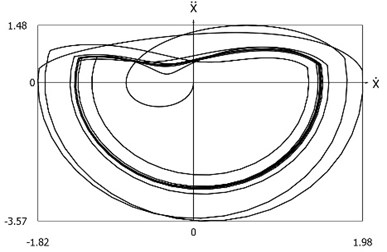b) Acceleration as function of velocity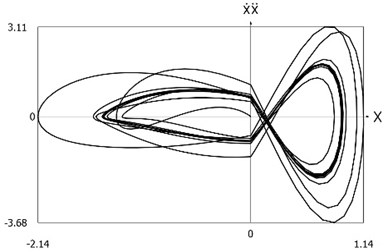c) Velocity multiplied by acceleration as function of displacement

Fig. 12Force of stiffness as function of displacement of the system with different values of stiffness for positive and negative displacements when ω=1, f= 1, h= 0.1, p1= 1, p2= 2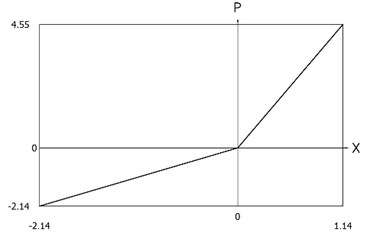## 5. Conclusions

Systems with different values of stiffness for positive and negative displacements are important in engineering applications. This change of the value of stiffness has an effect to the results of numerical calculation of dynamics of the system. For more precise investigation of dynamics a special numerical procedure is proposed in the paper. Numerical results for two values of time steps are presented without application of the proposed procedure and with application of it.

Displacement, velocity, acceleration, and velocity multiplied by acceleration as functions of time are investigated. Also phase trajectories of motion of the investigated system are presented. Force of stiffness as function of displacement is calculated and graphically represented.

From the presented results it is seen that the improved procedure for calculation of dynamics has advantages, which are seen from the comparison of corresponding graphical representations. This is especially evident from the comparison of corresponding drawings involving accelerations as well as from the comparison of representations of force of stiffness as function of displacement in the vicinity of the origin of the coordinate system. The presented graphical representations show the advantages of the improved procedure for calculation of dynamics of the system with different values of stiffness for positive and negative displacements.

The results of the performed investigation are applied in the process of design of elements of robots.